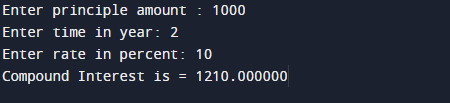# c program to calculate compound interest

In this tutorial you will learn about the c program to calculate compound interest and its application with practical example.

In this tutorial, we will learn to create a C program that  will Calculate Compound Interest    using C programming.

## Prerequisites

Before starting with this tutorial we assume that you are best aware of the following C programming topics:

• Arithmetic operators.
•  Basic input/output
• C loop statements
• Operator precedence
• Data types.

## What Is Compound Interest?

Compound interest (or compounding interest) is an interest to the principal sum of loan or deposit by consumer or by bank to consumer, or in other words,Compound Interest is interest on interest.

CI is interest earned from the original principal +  accumulated interest.

## formula for Compound Interest is.Here,

P is principle amount.
R is the rate in Percent.
T is time in year.

## OutputIn this tutorial we have learn about the c program to calculate compound interest and its application with practical example. I hope you will like this tutorial.